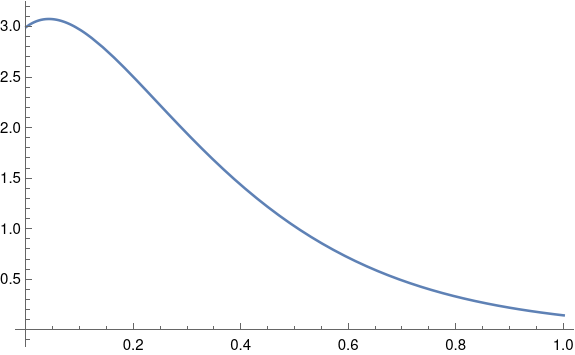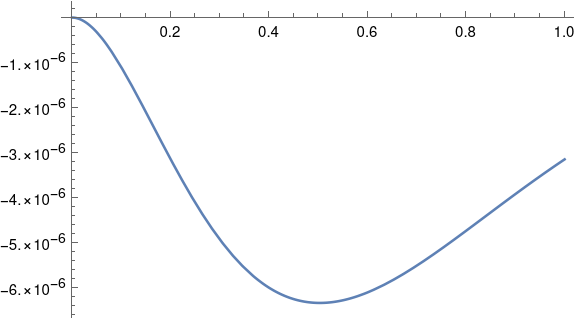# Double roots and ODEs

This post will resolve a sort of paradox. The process of solving a difference or differential equation is different when the characteristic equation has a double root. But intuitively there shouldn’t be much difference between having a double root and having two roots very close together.

I’ll first say how double roots effect finding solutions to difference and differential equations, then I’ll focus on the differential equations and look at the difference between a double root and two roots close together.

## Double roots

The previous post looked at a second order difference equation and a second order differential equation. Both are solved by analogous techniques. The first step in solving either the difference equation

ayn + byn-1 + cyn-2 = 0

or the differential equation

ay″ + by′ + cy = 0

is to find the roots of the characteristic equation

aλ² + bλ + c = 0.

If there are two distinct roots to the characteristic equation, then each gives rise to an independent solution to the difference or differential equation. The roots may be complex, and that changes the qualitative behavior of the solution, but it doesn’t change the solution process.

But what if the characteristic equation has a double root λ? One solution is found the same way: λn for the difference equation and exp(λt) for the differential equation. We know from general theorems that a second independent solution must exist, but how do we find it?

For the differential equation a second solution is nλn and for the differential equation t exp(λt) is our second solution.

## Close roots

For the rest of the post I’ll focus on differential equations, though similar remarks apply to difference equations.

If λ = 5 is a double root of our differential equation then the general solution is

α exp(5t) + β t exp(5t)

for some constants a and b. But if our roots are λ = 4.999 and λ = 5.001 then the general solution is

α exp(4.999 t) + β exp(5.001 t)

Surely these two solutions can’t be that different. If the parameters for the differential equation are empirically determined, can we even tell the difference between a double root and a pair of distinct roots a hair’s breadth apart?

On the other hand, β exp(5t) and β t exp(5t) are quite different for large t. If t = 100, the latter is 100 times larger! However, this bumps up against the Greek letter paradox: assuming that parameters with the same name in two equations mean the same thing. When we apply the same initial conditions to the two solutions above, we get different values of α and β for each, So comparing exp(5t) and t exp(5t) is the wrong comparison. The right comparison is between the solutions to two initial value problems.

We’ll look at two example, one with λ positive and one with λ negative.

## Example with λ > 0

If λ > 0, then solutions grow exponentially as t increases. The size of β t exp(λt) will eventually matter, regardless of how small β is. However, any error in the differential equation, such as measurement error in the initial conditions or error in computing the solution numerically, will also grow exponentially. The difference between a double root and two nearby roots may matter less than, say, how accurately the initial conditions are know.

Let’s look at

y″ – 10 y′ + 25 y = 0

and

y″ – 10 y′ + 24.9999 y = 0

both with initial conditions y(0) = 3 and y′(0) = 4.

The characteristic equation for the former has a double root λ = 5 and that of the latter has roots 4.99 and 5.01.

The solution to the first initial value problem is

3 exp(5t) – 11 t exp(5t)

and the solution to the second initial value problem is

551.5 exp(4.99t) – 548.5 exp(5.01t).

Notice that the coefficients are very different, illustrating the Greek letter paradox.

The expressions for the two solutions look different, but they’re indistinguishable for small t, say less than 1. But as t gets larger the solutions diverge. The same would be true if we solved the first equation twice, with y(0) = 3 and y(0) = 3.01.

## Example with λ < 0

Now let’s look at

y″ + 10 y′ + 25 y = 0

and

y″ + 10 y′ + 24.9999 y = 0

both with initial conditions y(0) = 3 and y′(0) = 4. Note that the velocity coefficient changed sign from -10 to 10.

Now the characteristic equation of the first differential equation has a double root at -5, and the second has roots -4.99 and -5.01.

The solution to the first initial value problem is

3 exp(-5t)  + 19 t exp(-5t)

and the solution to the second initial value problem is

951.5 exp(-4.99t) – 948.5 exp(5.01t).

Again the written forms of the two solutions look quite different, but their plots are indistinguishable.Unlike the case λ > 0, now with λ < 0 the difference between the solutions is bounded. Before the solutions started out close together and eventually diverged. Now the solutions are close together forever.

Here’s a plot of the difference between the two solutions.So the maximum separation between the two solutions occurs somewhere around t = 0.5 where the solutions differ in the sixth decimal place.

## Conclusion

Whether the characteristic equation has a double root or two close roots makes a significant difference in the written form of the solution to a differential equation. If the roots are positive, the solutions are initially close together then diverge. If the roots are negative, the solutions are always close together.

## One thought on “Double roots and ODEs”

1. 948.5 exp(5.01t) -> 948.5 exp(-5.01t)